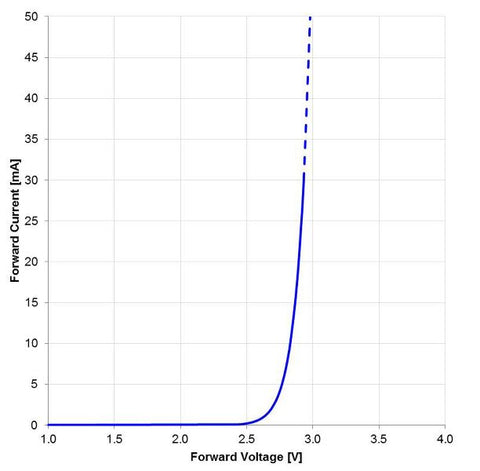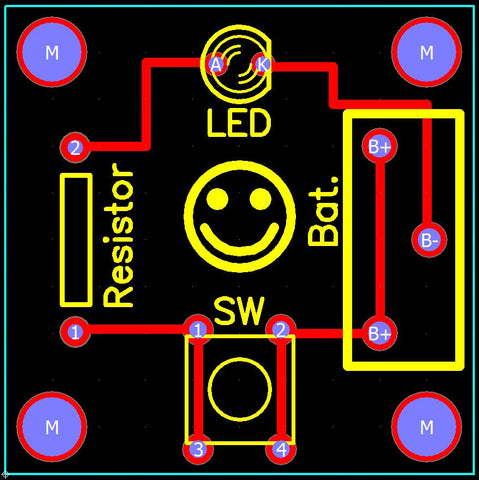# Understanding: Hello World Circuit Board

The Hello World board has four primary components:

1. Battery (Bat.)
2. Push Button Switch(SW)
3. Resistor
4. LED

The circuit works by pushing the button which completes the connection.  Electricity flows from the battery, through the LED, through the resistor, through the switch, and back to the battery.Hello World Schematic

LEDs have a Forward Voltage which is the amount of voltage drop or loss that occurs through the LED when operating at the nominal current load.  As current goes up, so does the Forward Voltage.  As current flow goes down, so does the Forward Voltage.  The graph below shows the relationship between the two.  Each LED type and brand is slightly different.  This graph applies for the type of LED used in the Hello World board.  At nominal current the LED in the board has a Forward Voltage of 2.8v.  ﻿Forward Current﻿ is the amount of current flowing at a given ﻿﻿Forward Voltage.Forward Voltage vs Forward Current

To calculate the current flow we need to use the equation, V = IR.  Where V is the voltage, I is the current, and R is the resistance.  Our resistance is known, a 47Ohm resistor.  Our voltage can be estimated by subtracting the Forward Voltage from the batteries voltage.  This works because of the relationship shown in the graph above, at different Forward Current levels the Forward Voltage is unvarying until you get to current draw of less than 5mA.  Assuming the battery has 3.3v when fully charged, our voltage will be 3.3v-2.8v = .5v.  Now we can calculate the current as .5v/47Ohms = ~10mA.

The layout of the Hello World printed circuit board(pcb) is shown below.  The red lines are the copper connections of the board.  If you compare the board layout to the schematic shown above you will see that they are the same.  Some things that you will notice that are different is the pushbutton had four leads on the board instead of the two shown in the schematic. The battery has three leads instead of two as well.  This is because the extra leads -- when soldered to the board -- are used for structural support.  This help prevents the pushbutton and battery mount from being broken off as they are handled by a person.Hello World Board Layout